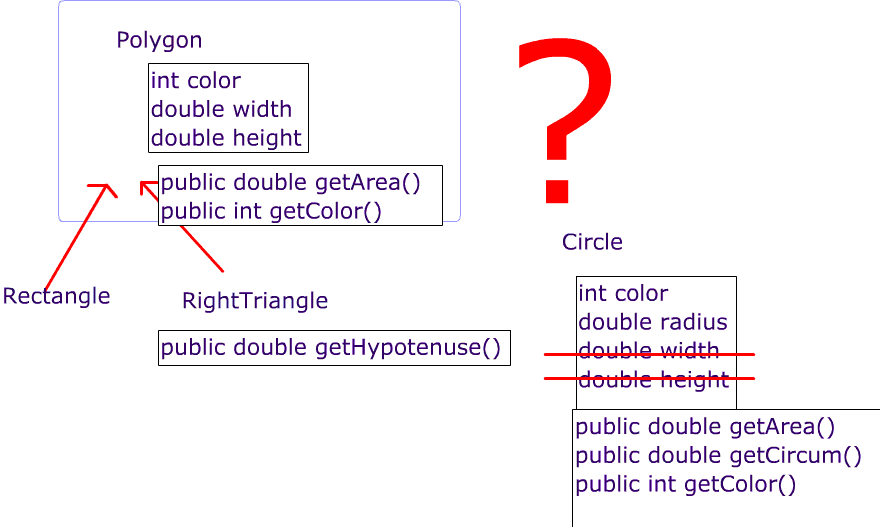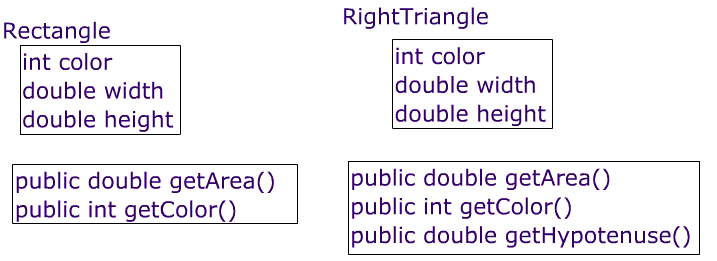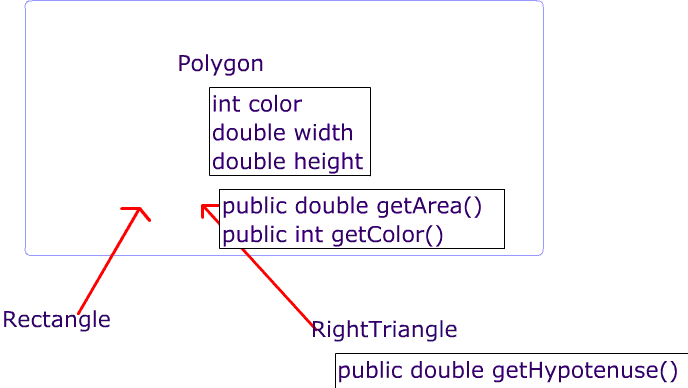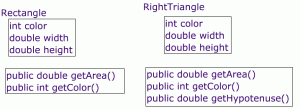# Circle Class

Create the following class

# Circle

• public Circle( double _radius, int _col)

instance Variables

• int color

Accesor Methods

• public double getArea(); //returns the area of the triangle
• public double getCircum() ;// returns the circumference

# What should Circle Extend?# What relationships exist between super and sub classes?

• How can we use superclasses to improve ourInteractiveMath2 code?
• To be implemented in  computeNetArea() method
• How can an element in the Polygon2 ‘ list that  is a RightTriangle2 call the getHypotenuse() method?
• To be implemented in the new method void printDetails() which should , among other , things print the hypotenuse length for any RightTriangle2’s?
• What is Inherited by subclasses?
• Constructors?
• instance variables
• static variables?

Next: What if we want to add a Circle Class

# SuperClasses are Great II

How can we improve our current class structure?We are going to create a second “Generation” of classes and will denote this change by appending a ‘2’ to the names of all classes in this “generation”### Class: Polygon2 (superclass of Triangle2, Rectangle2)  Polygon2Constructor

public Polygon2(int _col, double _width, double _height, int _sides)

instance Variables

• private int color;
• private int numSides
• protected double width;
• protected double height

Methods

• public int getColor()
• public double getWidth()
• public double getHeight()

### Class: Rectangle2 extends Polygon2  Rectangle2instance Variables

None needed (see superclass)

Accesor Methods //override each of the superclass

• public double getArea(); //returns the area of the triangle

### Class: RightTriangle2 extends Polygon2  RightTriangle2instance Variables

None needed (see superclass)

Accesor Methods //override each of the superclass

• public double getArea(); //returns the area of the triangle
• public double getHypotenuse()

Next: What is inherited?

# Question: What limitations seem to exist with our use of classes?

I. Implement all Methods of the Triangle and Rectangle methods belowClass: RectangleRectangle

Constructor

• public Rectangle ( int  _col,  double  _width,  double _height)

instance Variables

• private int color;
• private double width;
• private double height ;

Accessor Methods

• public double getArea(); //returns the area of the rectangle
• public int getColor()

### Class: RightTriangleRightTriangle

Constructor

• public RightTriangle( int _col,  double  _width,  double _height)

instance Variables

• private int color;
• private double width;
• private double height;

Accessor Methods

• public double getArea(); //returns the area of the triangle
• public int getColor()
• public double getHypotenuse() ;

### Class: InteractiveMath

instance Variables

• ArrayList<Rectangle> rectangles;
• ArrayList<RightTriangle> triangles;

Accesor Methods

• public double computeNetArea(){ //@ returns the area of the rectangles and triangles

Next  : Super Classes are great 2

Then  : constructors and inheritance.

after that : What is “Inherited” by Subclasses?

interactiveMath_arrays